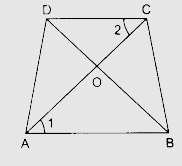# Diagonals of a trapezium ABCD with $AB \| DC$ intersect each other at the point O. If $AB = 2 CD$, find the ratio of the areas of triangles AOB and COD.

Given:

Diagonals of a trapezium ABCD with $AB \| DC$ intersect each other at the point O.

$AB = 2 CD$

To do:

We have to find the ratio of the areas of triangles AOB and COD.

Solution:In $\triangle \mathrm{AOB}$ and $\triangle \mathrm{COD}$,

$\angle \mathrm{AOB} =\angle \mathrm{COD}$       (vertically opposite angles)

$\angle 1=\angle 2$     (Alternate angles)

Therefore, by AA similarity,

$\Delta \mathrm{AOB} \sim \Delta \mathrm{COD}$

This implies,

$\frac{\operatorname{ar} \Delta \mathrm{AOB}}{\operatorname{ar} \Delta \mathrm{COD}}=\frac{\mathrm{AB}^{2}}{\mathrm{CD}^{2}}$

$=\frac{(2 \mathrm{CD})^{2}}{\mathrm{CD}^{2}}$

$=\frac{4 \mathrm{CD}^{2}}{\mathrm{CD}^{2}}$

$=\frac{4}{1}$

The ratio of the areas of triangles AOB and COD is $4:1$.